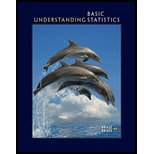Chapter 3, Problem 2LCWPUnderstanding Basic Statistics

8th Edition
Charles Henry Brase + 1 other
ISBN: 9781337558075

Solutions

Chapter
SectionUnderstanding Basic Statistics

8th Edition
Charles Henry Brase + 1 other
ISBN: 9781337558075
Textbook Problem

Why do we need to study the variation of a collection of data? Why isn’t the average by itself adequate? We have studied three ways to measure variation. The range, the standard deviation, and, to a large extent, a box-and-whisker plot all indicate the variation within a data collection. Discuss similarities and differences among these ways to measure data variation. Why would it seem reasonable to pair the median with a box-and-whisker plot and to pair the mean with the standard deviation? What are the advantages and disadvantages of each method of describing data spread? Comment on statements such as the following: (a) The range is easy to compute, but it doesn’t give much information; (b) although the standard deviation is more complicated to compute, it has some significant applications; (c) the box-and-whisker plot is fairly easy to construct, and it gives a lot of information at a glance.

To determine

To explain: The mean, median, and mode all represent averages in this context, discuss the differences among these averages, also explain the mean (a balance point), the median (a midway point), the mode (the most common data point), and list three areas of daily life describe an “average.”

Explanation

The central tendencies are the only way by which to know about the concentration of the observation. It is important to study the variation of data collection because it will help to understand the concepts of the collected data. It shows how the information is spread out through the data giving a better understanding of the material.

An average is an attempt to summarize a set of data using just one number. An average taken by itself may not always be very meaningful. It needs a statistical cross-reference that measures the spread of the data. Hence, the average by itself is not adequate.

A range is the most common and easily understandable measure of dispersion. It is the difference between two extreme observations of the data set. The standard deviation is a measure of how far the data points are spread out. It is least affected by the fluctuation of the observations. The standard deviation is zero if all the observations are constant. A box and whisker plot is an effective visual representation of both central tendency and dispersion. The “box” of the box plot shows the middle or “most typical” 50% of the values, while the “whiskers” of the box plot show the more extreme values.

A box-and-whisker plot is a quick way of showing the variability of a data set. It displays the range and distribution of data along the number line. A box and whisker plot also called a box plot displays the five-number summary of a set of data. The median is the middle point of a data set; 50% of the values are below this point, and 50% are above this point. The median or middle value splits the set of data into two equal numbered groups. The medians can be found throughout the boxes because of how each set of data is distributed. Outliers will make a difference. So it seems reasonable to pair the median with a box-and-whisker plot.

The standard deviation is a statistic that measures the dispersion of a dataset relative to its mean and is calculated as the square root of the variance. If the data points are further from the mean, there is a higher deviation within the data set; thus, the more spread out the data, the higher the standard deviation. So, it seems reasonable to pair the mean with the standard deviation.

Advantages of range, standard deviation, and box-and-whisker plot: Range is the simplest of measures of dispersion and easy to calculate and easy to understand...

Still sussing out bartleby?

Check out a sample textbook solution.

See a sample solution

The Solution to Your Study Problems

Bartleby provides explanations to thousands of textbook problems written by our experts, many with advanced degrees!

Get Started

Find f'(x) and f"(x). f(x)=xex

Single Variable Calculus: Early Transcendentals, Volume I

Solve the equations in Exercises 126. 14x2=0

Finite Mathematics and Applied Calculus (MindTap Course List)

In Exercises 17-20, determine whether the point lies on the graph of the function. 17. (2, 3); g(x)=x21

Applied Calculus for the Managerial, Life, and Social Sciences: A Brief Approach

In Exercises 2532, find the inverse of the matrix if it exist. A=

Finite Mathematics for the Managerial, Life, and Social Sciences